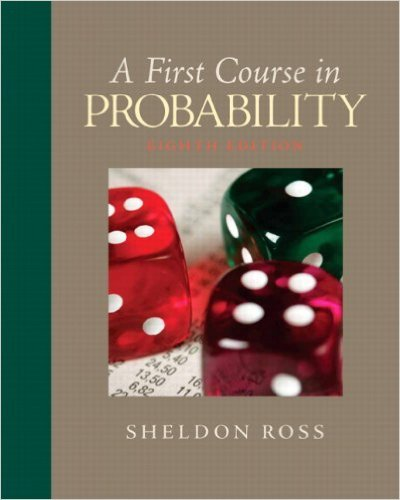×

×

# Solutions for Chapter 7: Properties of Expectation## Full solutions for First Course in Probability | 8th Edition

ISBN: 9780136033134Solutions for Chapter 7: Properties of Expectation

Solutions for Chapter 7
4 5 0 391 Reviews
23
3
##### ISBN: 9780136033134

Summary of Chapter 7: Properties of Expectation

Chapter 7: Properties of Expectation includes 79 full step-by-step solutions. This textbook survival guide was created for the textbook: First Course in Probability, edition: 8. Since 79 problems in chapter 7: Properties of Expectation have been answered, more than 26571 students have viewed full step-by-step solutions from this chapter. This expansive textbook survival guide covers the following chapters and their solutions. First Course in Probability was written by and is associated to the ISBN: 9780136033134.

Key Statistics Terms and definitions covered in this textbook
• 2 k factorial experiment.

A full factorial experiment with k factors and all factors tested at only two levels (settings) each.

• -error (or -risk)

In hypothesis testing, an error incurred by rejecting a null hypothesis when it is actually true (also called a type I error).

• Additivity property of x 2

If two independent random variables X1 and X2 are distributed as chi-square with v1 and v2 degrees of freedom, respectively, Y = + X X 1 2 is a chi-square random variable with u = + v v 1 2 degrees of freedom. This generalizes to any number of independent chi-square random variables.

• Alternative hypothesis

In statistical hypothesis testing, this is a hypothesis other than the one that is being tested. The alternative hypothesis contains feasible conditions, whereas the null hypothesis speciies conditions that are under test

• Analytic study

A study in which a sample from a population is used to make inference to a future population. Stability needs to be assumed. See Enumerative study

• Attribute control chart

Any control chart for a discrete random variable. See Variables control chart.

• Average run length, or ARL

The average number of samples taken in a process monitoring or inspection scheme until the scheme signals that the process is operating at a level different from the level in which it began.

• Chi-square test

Any test of signiicance based on the chi-square distribution. The most common chi-square tests are (1) testing hypotheses about the variance or standard deviation of a normal distribution and (2) testing goodness of it of a theoretical distribution to sample data

• Comparative experiment

An experiment in which the treatments (experimental conditions) that are to be studied are included in the experiment. The data from the experiment are used to evaluate the treatments.

• Conditional probability density function

The probability density function of the conditional probability distribution of a continuous random variable.

• Control chart

A graphical display used to monitor a process. It usually consists of a horizontal center line corresponding to the in-control value of the parameter that is being monitored and lower and upper control limits. The control limits are determined by statistical criteria and are not arbitrary, nor are they related to speciication limits. If sample points fall within the control limits, the process is said to be in-control, or free from assignable causes. Points beyond the control limits indicate an out-of-control process; that is, assignable causes are likely present. This signals the need to ind and remove the assignable causes.

• Convolution

A method to derive the probability density function of the sum of two independent random variables from an integral (or sum) of probability density (or mass) functions.

• Cumulative normal distribution function

The cumulative distribution of the standard normal distribution, often denoted as ?( ) x and tabulated in Appendix Table II.

• Deming’s 14 points.

A management philosophy promoted by W. Edwards Deming that emphasizes the importance of change and quality

• Error propagation

An analysis of how the variance of the random variable that represents that output of a system depends on the variances of the inputs. A formula exists when the output is a linear function of the inputs and the formula is simpliied if the inputs are assumed to be independent.

• Exponential random variable

A series of tests in which changes are made to the system under study

• Fixed factor (or fixed effect).

In analysis of variance, a factor or effect is considered ixed if all the levels of interest for that factor are included in the experiment. Conclusions are then valid about this set of levels only, although when the factor is quantitative, it is customary to it a model to the data for interpolating between these levels.

• Fraction defective

In statistical quality control, that portion of a number of units or the output of a process that is defective.

• Generator

Effects in a fractional factorial experiment that are used to construct the experimental tests used in the experiment. The generators also deine the aliases.

• Goodness of fit

In general, the agreement of a set of observed values and a set of theoretical values that depend on some hypothesis. The term is often used in itting a theoretical distribution to a set of observations.